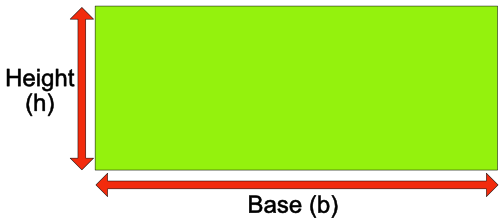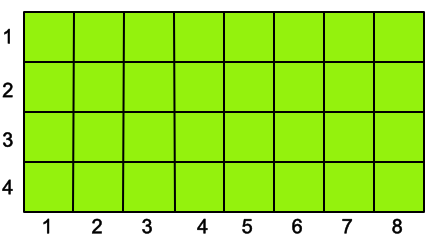Area of a Rectangle

## Area of a Rectangle

The area of a rectangle is given by: A = bhwhere A is the area, b = base length and h is the height.

A rectangle that is 8 units along its base length, and 4 units high, has an area of 32 units square.Area units are squared: for example, a rectangle of 5 cm x 3 cm has an area of 15 cm2.

## Example 1

What is the area of a rectangle with a base length of 15.5 cm and a height of 4 cm?

 Area = base x height = 15.5 x 4 = 62 cm2

Remember the answer is in cm2